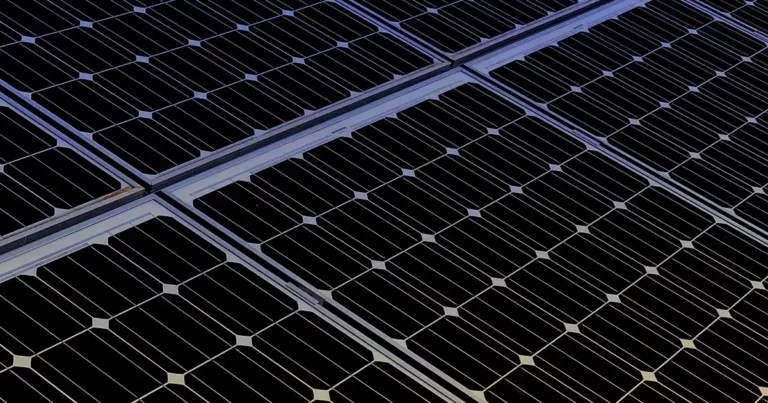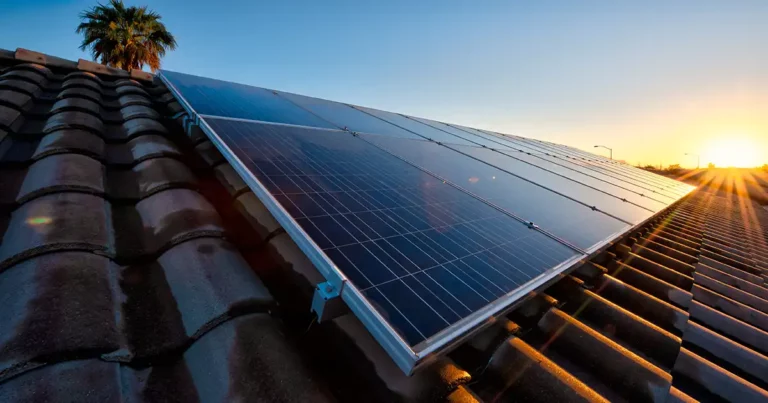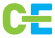# Calculating Solar Panels Efficiency for home

Introduction

As the world pivots towards sustainable energy solutions, understanding the efficiency of solar modules is pivotal for harnessing the full potential of solar power.

In this blog post, we will delve into the intricacies of calculating the efficiency of solar modules, exploring key concepts such as surface area, solar panel efficiency, solar cell efficiency, and the overall factors contributing to efficient solar energy generation.

Defining Solar Module Efficiency:

Understanding Efficiency: Solar module efficiency refers to the ratio of usable electrical power output to the amount of solar energy absorbed by the module. It is a critical metric in evaluating the performance of solar panels.

Expression: Efficiency (%) = (Usable Power Output / Solar Energy Input) x 100

Understanding Solar Panel Efficiency:

Solar Panel Efficiency: This is a measure of how well a solar panel converts sunlight into electricity. It is influenced by the efficiency of individual solar cells, as well as other factors such as reflectance losses and temperature effects.

Calculation: Solar Panel Efficiency (%) = (Usable Power Output of the Panel / Solar Energy Input) x 100

Solar Cell Efficiency:

Solar Cell Efficiency: At the heart of panels are solar cells, and their efficiency is a crucial factor. Solar cell efficiency is the percentage of sunlight that a solar cell converts into electricity.

Expression: Solar Cell Efficiency (%) = (Usable Power Output of the Cell / Solar Energy Input) x 100

Surface Area and Solar Module Efficiency:

Surface Area Impact: The total surface area of solar modules plays a significant role in determining their efficiency. Larger surface areas allow for the absorption of more sunlight, contributing to higher efficiency.

Influence on Efficiency: Solar Module Efficiency (%) = (Usable Power Output of the Module / (Solar Energy Input x Surface Area)) x 100

Factors Influencing Efficient Solar Energy Generation:

Angle of Incidence: The angle at which sunlight strikes the solar panel affects the efficiency. Adjusting the tilt of the panel to match the sun’s angle optimizes energy absorption.

Temperature Effects: Solar panels perform best at lower temperatures. Higher temperatures can reduce efficiency, making proper ventilation and cooling crucial.

Cleanliness: Dust, dirt, and other contaminants on the surface of solar panels can reduce their efficiency by blocking sunlight. Regular cleaning ensures optimal performance.

Measuring Usable Power Output:

Direct Current (DC) Output: Solar panels produce DC electricity. The usable power output is the DC power generated by the solar panel, which is then converted to AC for use in homes and businesses.

Power Output Measurement: Usable Power Output is usually measured in watts (W) or kilowatts (kW).

Solar Panel Testing Protocols:

Standard Test Conditions (STC): Solar panel efficiency is often tested under standard conditions, including an irradiance of 1000 watts per square meter and a temperature of 25 degrees Celsius.

Performance Ratio: The performance ratio is a measure of how well a solar panel performs under actual operating conditions, factoring in real-world variables.

Optimizing for Efficient Solar Energy:

Technology Advances: Stay abreast of technological advancements in solar panel technology, as newer models often feature higher efficiencies.

Regular Maintenance: Periodic checks, cleaning, and maintenance ensure that solar panels operate at peak efficiency.

Professional Assessment: Engage professionals for periodic assessments to identify and address any efficiency-reducing issues promptly.

Example Calculation

Let’s consider a hypothetical scenario:

• Solar Panel Efficiency: 18%
• Surface Area of the Panel: 2 square meters
• Solar Energy Input: 1000 watts per square meter
• Usable Power Output of the Panel: 250 watts

Solar Module Efficiency:

Efficiency (%)=(250 watts/1000 watts/sqm×2 sqm)×100≈12.5%

Solar Panel Efficiency:

Solar Panel Efficiency (%)=(250 watts/1000 watts)×100=25%

Solar Cell Efficiency: In this scenario, let’s assume the solar cell efficiency is the same as the panel efficiency.

Solar Cell Efficiency=25%

Conclusion

In this example, the overall solar module efficiency is 12.5%, the solar panel efficiency is 25%, and the solar cell efficiency is also 25%. Understanding these values provides insights into the performance of the solar module in converting sunlight into usable electricity. Real-world efficiencies can vary, and advancements in technology continually contribute to improving these values for more efficient solar energy systems. By mastering these calculations and concepts, you can make informed decisions and contribute to a more sustainable future powered by efficient solar energy.

## Check out more blogsA Comprehensive Guide to Solar Panels for Home Use...Solar Energy in Sports Stadiums in India: A Greener...Solar-Powered Public Transportation: Case Study in...The Rise of Mono PERC: A Game-Changer in Solar Energy...Solar Energy and the Fight Against Air Pollution Introduction...